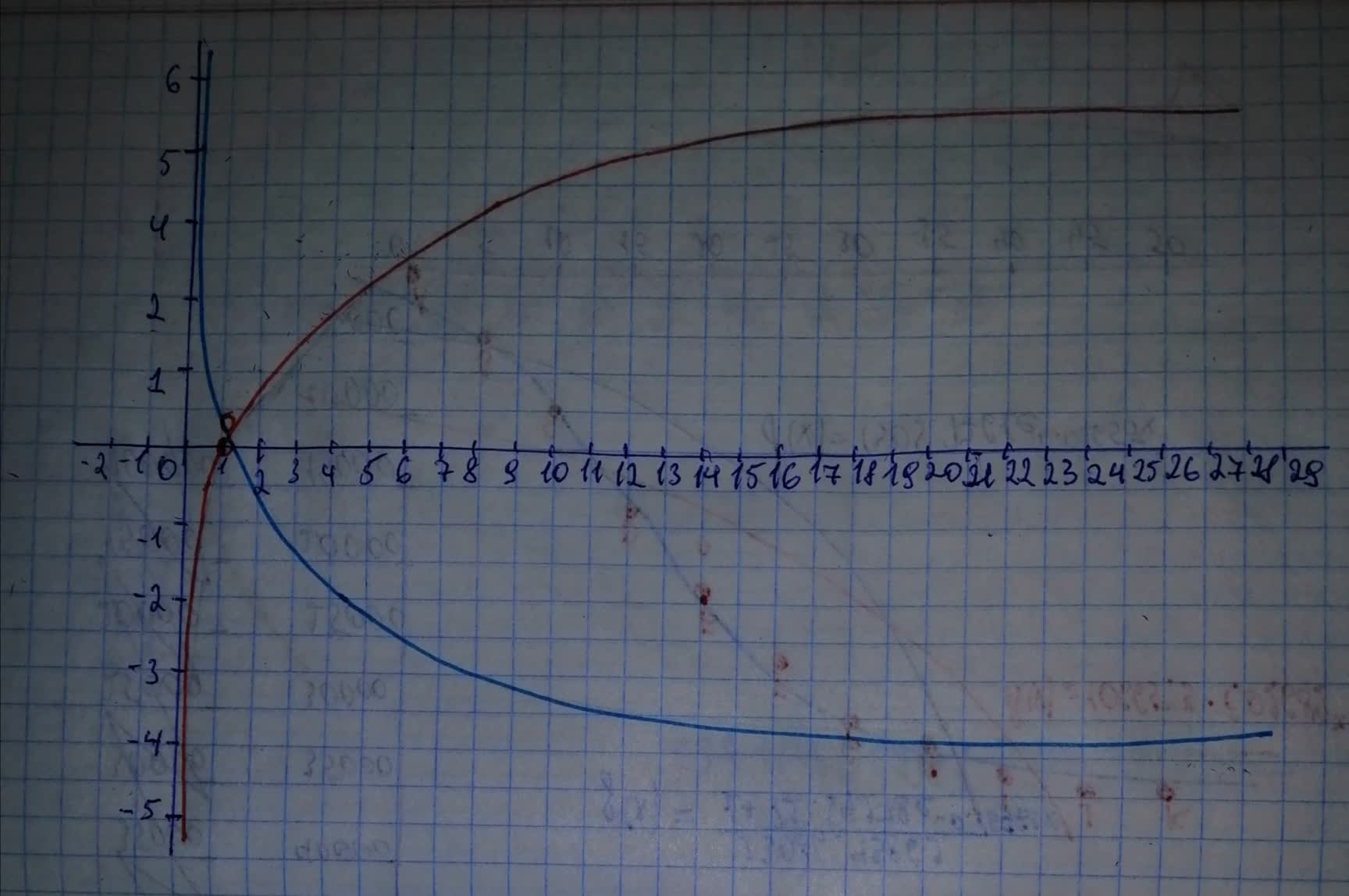Question# For y= -log_{2}x. a) Use transformations of the graphs of y=log_{2}x and y=log_{3}x o graph the given functions. b) Write the domain and range in interval notation. c) Write an equation of the asymptote.

Transformations of functions
ANSWEREDFor $$\displaystyle{y}=\ -{{\log}_{{{2}}}{x}}$$.
a) Use transformations of the graphs of $$\displaystyle{y}={{\log}_{{{2}}}{x}}$$ and $$\displaystyle{y}={{\log}_{{{3}}}{x}}$$ o graph the given functions.
b) Write the domain and range in interval notation.
c) Write an equation of the asymptote.2021-02-12
Step 1
a) Start from the graph of the parent function $$\displaystyle{f{{\left({x}\right)}}}={{\log}_{{{2}}}{x}}$$.
As we can see the given function $$\displaystyle{y}=\ -{{\log}_{{{2}}}{x}}$$ can be expressed in terms of the parent function f as $$\displaystyle{y}=\ -{f{{\left({x}\right)}}}$$
This indicates that the graph of the function $$\displaystyle{y}=\ -{{\log}_{{{2}}}{x}}$$ will be the same as the graph of the parent function $$\displaystyle{f{{\left({x}\right)}}}={{\log}_{{{2}}}{x}}$$ reflected through the x-axis.
See the graphs in the picture below:Step 2
b) The domain of the function $$\displaystyle{y}=\ -{{\log}_{{{2}}}{x}}$$ is the interval: $$\displaystyle{\left({0},\ +\infty\right)}$$
The range of the function $$\displaystyle{y}=\ -{{\log}_{{{2}}}{x}}$$ is the interval $$\displaystyle{\left(-\infty,\ +\infty\right)}$$
c) The vertical asymptote of the graph of this function is the line $$\displaystyle{x}={0}$$